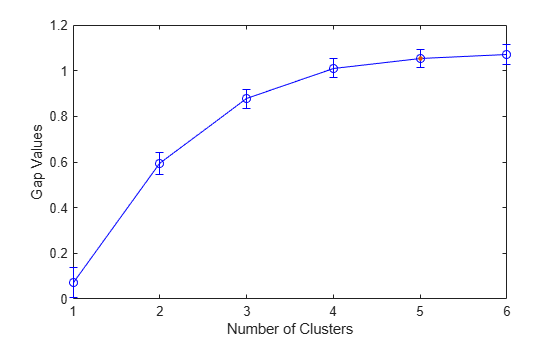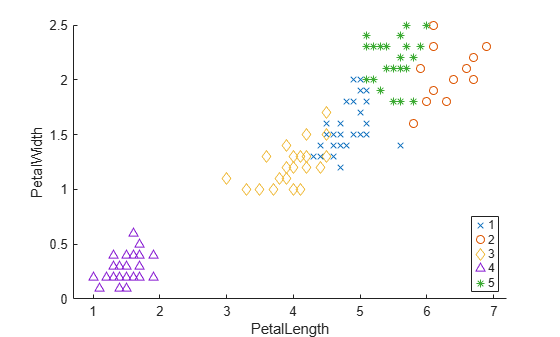Main Content

# GapEvaluation

Package: clustering.evaluation
Superclasses: `ClusterCriterion`

Gap criterion clustering evaluation object

## Description

`GapEvaluation` is an object consisting of sample data, clustering data, and gap criterion values used to evaluate the optimal number of clusters. Create a gap criterion clustering evaluation object using `evalclusters`.

## Construction

`eva = evalclusters(x,clust,'Gap')` creates a gap criterion clustering evaluation object.

`eva = evalclusters(x,clust,'Gap',Name,Value)` creates a gap criterion clustering evaluation object using additional options specified by one or more name-value pair arguments.

### Input Arguments

expand all

Input data, specified as an N-by-P matrix. N is the number of observations, and P is the number of variables.

Data Types: `single` | `double`

Clustering algorithm, specified as one of the following.

 `'kmeans'` Cluster the data in `x` using the `kmeans` clustering algorithm, with `'EmptyAction'` set to `'singleton'` and `'Replicates'` set to `5`. `'linkage'` Cluster the data in `x` using the `clusterdata` agglomerative clustering algorithm, with `'Linkage'` set to `'ward'`. `'gmdistribution'` Cluster the data in `x` using the `gmdistribution` Gaussian mixture distribution algorithm, with `'SharedCov'` set to `true` and `'Replicates'` set to `5`.

If `criterion` is `'CalinskiHarabasz'`, `'DaviesBouldin'`, or `'silhouette'`, you can specify a clustering algorithm using a function handle. The function must be of the form `C = clustfun(DATA,K)`, where `DATA` is the data to be clustered, and `K` is the number of clusters. The output of `clustfun` must be one of the following:

• A vector of integers representing the cluster index for each observation in `DATA`. There must be `K` unique values in this vector.

• A numeric n-by-K matrix of score for n observations and K classes. In this case, the cluster index for each observation is determined by taking the largest score value in each row.

If `criterion` is `'CalinskiHarabasz'`, `'DaviesBouldin'`, or `'silhouette'`, you can also specify `clust` as a n-by-K matrix containing the proposed clustering solutions. n is the number of observations in the sample data, and K is the number of proposed clustering solutions. Column j contains the cluster indices for each of the N points in the jth clustering solution.

Data Types: `single` | `double` | `char` | `string` | `function_handle`

Name-Value Pair Arguments

Specify optional comma-separated pairs of `Name,Value` arguments. `Name` is the argument name and `Value` is the corresponding value. `Name` must appear inside quotes. You can specify several name and value pair arguments in any order as `Name1,Value1,...,NameN,ValueN`.

Example: `'KList',[1:5],'Distance','cityblock'` specifies to test 1, 2, 3, 4, and 5 clusters using the city block distance metric.

Number of reference data sets generated from the reference distribution `ReferenceDistribution`, specified as the comma-separated pair consisting of `'B'` and a positive integer value.

Example: `'B',150`

Data Types: `single` | `double`

Distance metric used for computing the criterion values, specified as the comma-separated pair consisting of `'Distance'` and one of the following.

 `'sqEuclidean'` Squared Euclidean distance `'Euclidean'` Euclidean distance `'cityblock'` Sum of absolute differences `'cosine'` One minus the cosine of the included angle between points (treated as vectors) `'correlation'` One minus the sample correlation between points (treated as sequences of values)

For detailed information about each distance metric, see `pdist`.

You can also specify a function for the distance metric by using a function handle. The distance function must be of the form

`d2 = distfun(XI,XJ),`
where `XI` is a 1-by-n vector corresponding to a single row of the input matrix `X`, and `XJ` is an m2-by-n matrix corresponding to multiple rows of `X`. `distfun` must return an m2-by-1 vector of distances `d2`, whose kth element is the distance between `XI` and `XJ(k,:)`.

`Distance` only accepts a function handle if the clustering algorithm `clust` accepts a function handle as the distance metric. For example, the `kmeans` clustering algorithm does not accept a function handle as the distance metric. Therefore, if you use the `kmeans` algorithm and then specify a function handle for `Distance`, the software errors.

• When `clust` is `'kmeans'` or `'gmdistribution'`, `evalclusters` uses the distance metric specified for `Distance` to cluster the data.

• If `clust` is `'linkage'`, and `Distance` is either `'sqEuclidean'` or `'Euclidean'`, then the clustering algorithm uses Euclidean distance and Ward linkage.

• If `clust` is `'linkage'` and `Distance` is any other metric, then the clustering algorithm uses the specified distance metric and average linkage.

• In all other cases, the distance metric specified for `Distance` must match the distance metric used in the clustering algorithm to obtain meaningful results.

Example: `'Distance','Euclidean'`

Data Types: `single` | `double` | `char` | `string` | `function_handle`

List of number of clusters to evaluate, specified as the comma-separated pair consisting of `'KList'` and a vector of positive integer values. You must specify `KList` when `clust` is a clustering algorithm name or a function handle. When `criterion` is `'gap'`, `clust` must be a character vector, a string scalar, or a function handle, and you must specify `KList`.

Example: `'KList',[1:6]`

Data Types: `single` | `double`

Reference data generation method, specified as the comma-separated pair consisting of `'ReferenceDistributions'` and one of the following.

 `'PCA'` Generate reference data from a uniform distribution over a box aligned with the principal components of the data matrix `x`. `'uniform'` Generate reference data uniformly over the range of each feature in the data matrix `x`.

Example: `'ReferenceDistribution','uniform'`

Method for selecting the optimal number of clusters, specified as the comma-separated pair consisting of `'SearchMethod'` and one of the following.

 `'globalMaxSE'` Evaluate each proposed number of clusters in `KList` and select the smallest number of clusters satisfying `$\text{Gap}\left(K\right)\ge GAPMAX-\text{SE}\left(GAPMAX\right),$`where K is the number of clusters, Gap(K) is the gap value for the clustering solution with K clusters, GAPMAX is the largest gap value, and SE(GAPMAX) is the standard error corresponding to the largest gap value. `'firstMaxSE'` Evaluate each proposed number of clusters in `KList` and select the smallest number of clusters satisfying `$\text{Gap}\left(K\right)\ge \text{Gap}\left(K+1\right)-\text{SE}\left(K+1\right),$`where K is the number of clusters, Gap(K) is the gap value for the clustering solution with K clusters, and SE(K + 1) is the standard error of the clustering solution with K + 1 clusters.

Example: `'SearchMethod','globalMaxSE'`

## Properties

 `B` Number of data sets generated from the reference distribution, stored as a positive integer value. `ClusteringFunction` Clustering algorithm used to cluster the input data, stored as a valid clustering algorithm name or function handle. If the clustering solutions are provided in the input, `ClusteringFunction` is empty. `CriterionName` Name of the criterion used for clustering evaluation, stored as a valid criterion name. `CriterionValues` Criterion values corresponding to each proposed number of clusters in `InspectedK`, stored as a vector of numerical values. `Distance` Distance metric used for clustering data, stored as a valid distance metric name. `ExpectedLogW` Expectation of the natural logarithm of W based on the generated reference data, stored as a vector of scalar values. W is the within-cluster dispersion computed using the distance metric `Distance`. `InspectedK` List of the number of proposed clusters for which to compute criterion values, stored as a vector of positive integer values. `LogW` Natural logarithm of W based on the input data, stored as a vector of scalar values. W is the within-cluster dispersion computed using the distance metric `Distance`. `Missing` Logical flag for excluded data, stored as a column vector of logical values. If `Missing` equals `true`, then the corresponding value in the data matrix `x` is not used in the clustering solution. `NumObservations` Number of observations in the data matrix `X`, minus the number of missing (`NaN`) values in `X`, stored as a positive integer value. `OptimalK` Optimal number of clusters, stored as a positive integer value. `OptimalY` Optimal clustering solution corresponding to `OptimalK`, stored as a column vector of positive integer values. If the clustering solutions are provided in the input, `OptimalY` is empty. `ReferenceDistribution` Reference data generation method, stored as a valid reference distribution name. `SE` Standard error of the natural logarithm of W with respect to the reference data for each number of clusters in `InspectedK`, stored as a vector of scalar values. W is the within-cluster dispersion computed using the distance metric `Distance`. `SearchMethod` Method for determining the optimal number of clusters, stored as a valid search method name. `StdLogW` Standard deviation of the natural logarithm of W with respect to the reference data for each number of clusters in `InspectedK`. W is the within-cluster dispersion computed using the distance metric `Distance`. `X` Data used for clustering, stored as a matrix of numerical values.

## Methods

 increaseB Increase reference data sets

### Inherited Methods

 addK Evaluate additional numbers of clusters compact Compact clustering evaluation object plot Plot clustering evaluation object criterion values

## Examples

collapse all

Evaluate the optimal number of clusters using the gap clustering evaluation criterion.

Load the sample data.

`load fisheriris`

The data contains sepal and petal measurements from three species of iris flowers.

Evaluate the number of clusters based on the gap criterion values. Cluster the data using `kmeans`.

```rng('default'); % For reproducibility eva = evalclusters(meas,'kmeans','gap','KList',[1:6])```
```eva = GapEvaluation with properties: NumObservations: 150 InspectedK: [1 2 3 4 5 6] CriterionValues: [0.0720 0.5928 0.8762 1.0114 1.0534 1.0720] OptimalK: 5 ```

The `OptimalK` value indicates that, based on the gap criterion, the optimal number of clusters is five.

Plot the gap criterion values for each number of clusters tested.

`plot(eva)`Based on the plot, the maximum value of the gap criterion occurs at six clusters. However, the value at five clusters is within one standard error of the maximum, so the suggested optimal number of clusters is five.

Create a grouped scatter plot to examine the relationship between petal length and width. Group the data by suggested clusters.

```figure PetalLength = meas(:,3); PetalWidth = meas(:,4); ClusterGroup = eva.OptimalY; gscatter(PetalLength,PetalWidth,ClusterGroup,'rbgkc','xod^*');```The plot shows cluster 4 in the lower-left corner, completely separated from the other four clusters. Cluster 4 contains flowers with the smallest petal widths and lengths. Cluster 2 is in the upper-right corner and contains flowers with the largest petal widths and lengths. Cluster 5 is next to cluster 2 and contains flowers with similar petal widths as the flowers in cluster 2, but smaller petal lengths than the flowers in cluster 2. Clusters 1 and 3 are near the center of the plot and contain flowers with measurements between the extremes.

expand all

## References

 Tibshirani, R., G. Walther, and T. Hastie. “Estimating the number of clusters in a data set via the gap statistic.” Journal of the Royal Statistical Society: Series B. Vol. 63, Part 2, 2001, pp. 411–423.

Download ebook